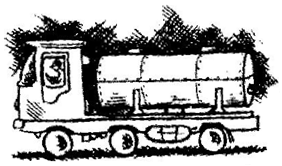# A milk tank is in the form of cylinder whose radius is 1.5 m and length is 7 m. Find the quantity of milk in liters that can be stored in the tank.Given

The radius of a cylindrical tank, r = 1.5 m

Height of cylindrical tank, h = 7 m

Find out

We have to find out the

the quantity of milk in litres that can be stored in the tank.

Solution

Volume of cylindrical tank, V = πr2h

= (22/7)×1.5×1.5 ×7

= 49.5 cm3

= 49.5×1000 liters = 49500 liters

[∵ 1 m3= 1000 liters]

Hence, required quantity of milk is 49500 liters.